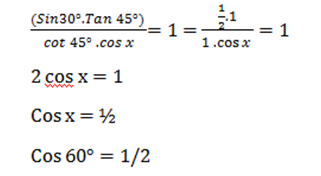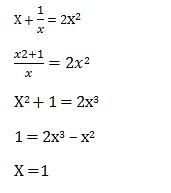# RRB ALP 2018 Practice Test Papers | Arithmetic Questions (Day-57)

Dear Aspirants, Here we have given the Important RRB ALP & Technicians Exam 2018 Practice Test Papers. Candidates those who are preparing for RRB ALP 2018 can practice these Arithmetic Questions to get more confidence to Crack RRB 2018 Examination.

[WpProQuiz 2767]

Click “Start Quiz” to attend these Questions and view Solutions

1). Simplify

529÷23 × 391 ÷17 ÷23

1. 529
2. 391
3. 17
4. 23

2). 16 Men can do a piece of work in 20 days. 20 women can do a piece of work in 10 days. If both they work together the work will finish in how many days?

1. 2 days
2. 3/4 days
3. 6 2/ 3 days
4. 10/3 days

3). A dishonest shopkeeper marked his goods price at 20 % profit and also he reduced 10 % of the quantity of product’s net weight. Finally how much % he gained?

1. 16%
2. 35 %
3. c.33 1/3%
4. 47%

4). Find the value of x

70% of 30 % of 60% of x = 126× 3

1. 1000
2. 5000
3. 3000
4. 2000

5). Area of Square is 16cm2. Find the Diagonal of the Square?

1. 2
2. 16
3. 4√2
4. 2√2

6). Age of A is twice as C, thrice as B. Total ages of A, B & C = 44. Find the A’s Age?

1. 20
2. 8
3. 24
4. 28

7). Area of Triangle is 48, what is the height of the triangle is the base of the triangle is 6 cm?

1. 6 cm
2. 16 cm
3. 8 cm
4. 12 cm

8). Find the value of x

(Sin30°. Tan 45°)/(cot 45° . cos x) = 1

1. 30°
2. 60°
3. 45°

9). Average of 11 observations is 16. Average of first 4 observations is 16, Last 5 observation is 18. Find the average value of rest of the numbers?

1. 10
2. 16
3. 11
4. 19

10). A number added with its reciprocal is equal to the double the square value of same number. Find the Number.

1. 4
2. 2
3. 1
4. 0

529 ×1/23 ×391 ×1/17 ×1/23 = 23

Given

16 Men ——- 20 days

20 women —- 10 days

16 M + 20 W =  ?

16 * 20 M= 20 * 10 W

8 M = 5 W => M = 5/8 W

16 (5/8) + 20 W =  ?

=>10 W + 20 W = 30 W

20 W ——- 10 days

30 W ——— ?

20×10/30 =  20/3  days=6  2/3

Let us consider CP of 100 g is Rs.100

Selling price at 20% profit= Rs. 120

Dishonest seller sells 90% of it, So CP in this transaction is Rs.90

Profit %= (120-90)/90×100=30/90×100=33 1/3%

(70/100) × (30/100) × (60/100) x = 126 × 3

X = 3000

Diagonal of a Square is = √2 a

Area = a2 = 16

Side = 4 cm

Diagonal = 4√2

A : B : C

6 : 2 : 3

(44 × 6) / 11 = 24

Area of Triangle = 1/2 × Base ×Height

48 = 1/2 × 6 × Height

H = 16cmTotal value = 11 ×16 = 154

Sum of first 4 value = 4 × 16 = 64

Sum of next 5 numbers = 5 ×18 = 90

Sum of those 9 value is = 64 + 90 = 154

Sum of Rest of the value = 176 – 154 = 22

Averages = 22 /2 = 11